# ISEE Middle Level Math : How to find the area of a square

## Example Questions

← Previous 1 3 4

### Example Question #26 : Squares

Which of the following is equal to the area of a square with perimeter 8 meters?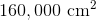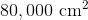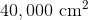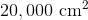Explanation:

The sidelength of a square is one-fourth its perimeter, so the sidelength here is one-fourth of 8, or 2, meters. One meter is equal to 100 centimeters, so the sidelength is 200 centimeters. Square this to get the area: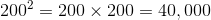square centimeters.

### Example Question #27 : Squares

Which of the following is the area of a square with perimeter 7 feet?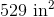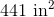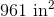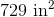Explanation:

Convert  7 feet to inches by multiplying by 12: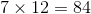inches.

The sidelength of a square is one-fourth its perimeter, so the sidelength here is one-fourth of 84 inches. This is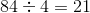inches.

Square this to get the area: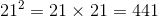square inches

### Example Question #28 : Squares

What is the area of a square with a side length of 4?

4

14

8

16

12

16

Explanation:

The area of a square is represented by the equation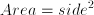.

Therefore the area of this square is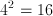.

### Example Question #29 : Squares

Square A has sides measuring 5 meters.  A second square, Square B, has sides that are 2 meters longer than the sides of Square A.  What is the difference in area of Square A and Square B?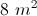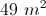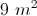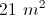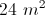Explanation:

The area of Square A is 5 * 5, or 25 m2.

Since each of Square B's sides is 2 meters longer, the sides measure 7 meters. Therefore, the area of square B is 49 m2.

Subtract to find the difference in areas: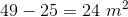### Example Question #30 : Squares

The ratio of the length of a side of one square to the length of the side of another square is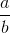. Give the ratio of the area of the second square to the area of the first square.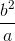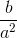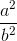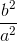Explanation:

The area of a square can be found as follows: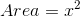Where: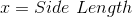So we can write: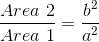### Example Question #31 : Squares

What is the area of a square if the length of one side is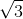?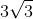Explanation:

The area of a square is found by multiplying one side by itself.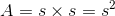We are given the side length, allowing us to solve.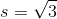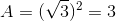### Example Question #32 : Squares

A square has an area of 36, and each side is equal toWhat is the value of?Explanation:

Each side of a square is equal to the square root of the area. The square root of 36 is 6, so each side is 6. Thus, 3x (being a side of the square is equal to 6. This means that x is equal to 2 because 3 times 2 is 6.

### Example Question #33 : Squares

Find the area of a square with side length 10.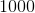Explanation:

To find the area of a square, simply use the formula. Thus,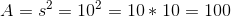### Example Question #34 : Squares

The area of a square is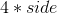?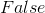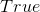Explanation:

The area of a square is the side length squared not the side length times.

### Example Question #35 : Squares

If you are given one side length of a square, you can find the area with that information.

True

False

To find the area of a square, you multiple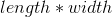.  But with a square all the sides are equal so the equation really is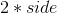or the side length squared.  Since you are given the side length, you can find the area.